# 题目详情

## 题干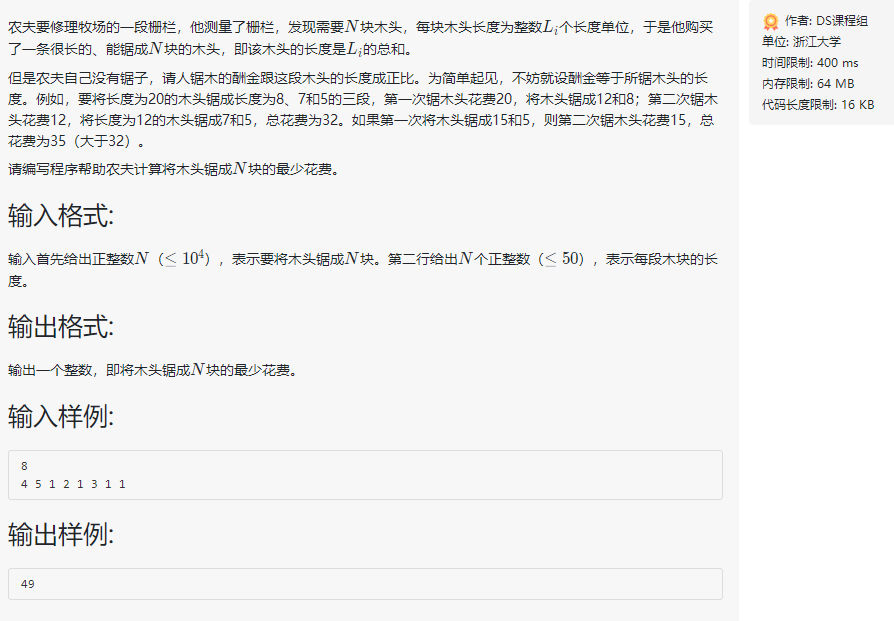## 输入样例

8
4 5 1 2 1 3 1 1


## 输出样例

49


# 哈夫曼树

## 情景模拟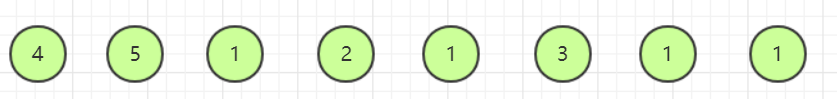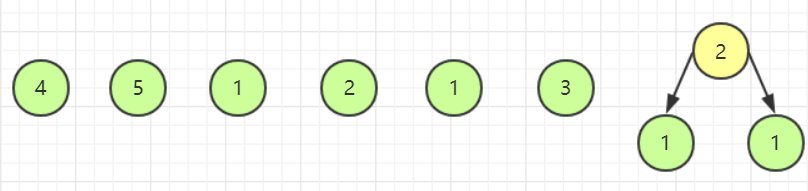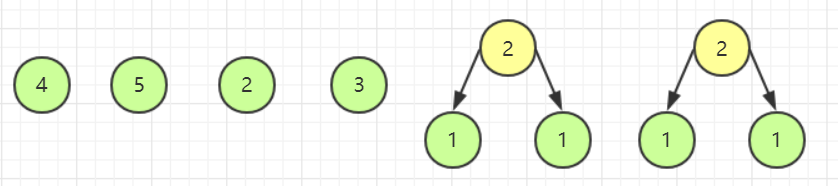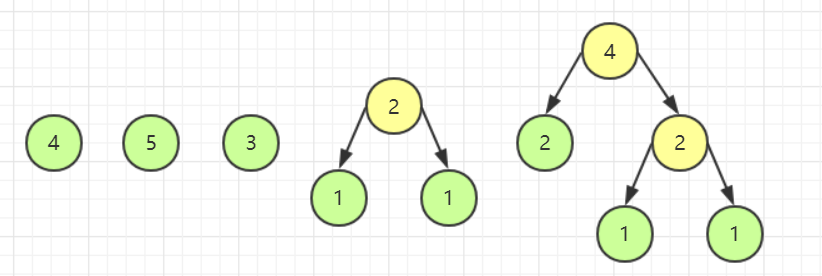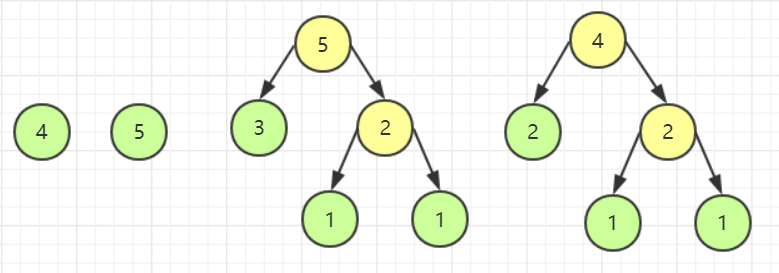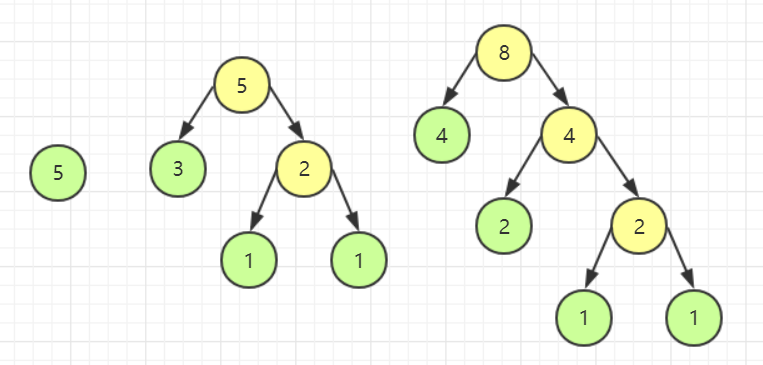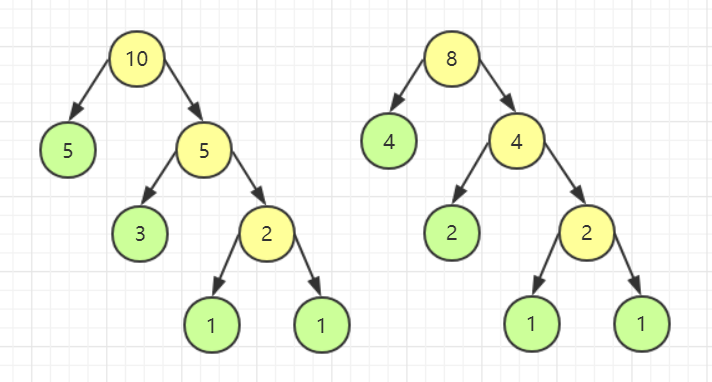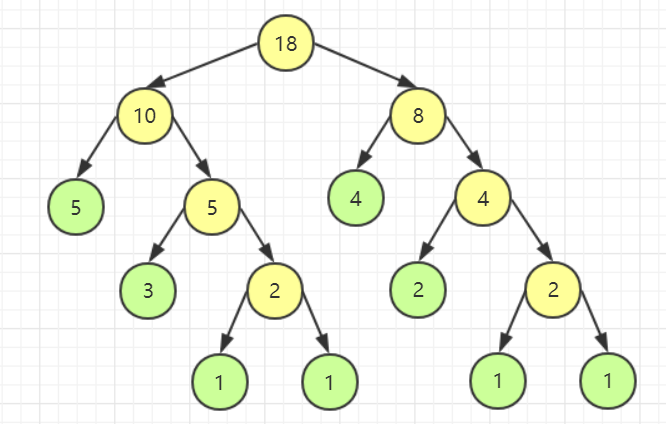## 代码实现

#include<iostream>
using namespace std;

typedef struct {
int weight;    //权值
int parent, lchild, rchild;    //指向双亲、左右孩子结点的游标
}HTNode, * HuffmanTree;
void CreatHuffmanTree(HuffmanTree& HT, int n);    //建哈夫曼树
void Select(HuffmanTree HT, int k, int &idx1, int &idx2);    //找孩子结点

int main()
{
int n;
HuffmanTree HT;
int sum = 0;

cin >> n;
CreatHuffmanTree(HT, n);    //建哈夫曼树
for (int i = n + 1; i < 2 * n; i++)    //算总花费
{
sum += HT[i].weight;
}
cout << sum;
}

void CreatHuffmanTree(HuffmanTree& HT, int n)
{
int m = 2 * n - 1;
int idx1, idx2;
int i;

if (n < 0)    //树的结点数为 0，即空树
return;
//二叉树初始化
HT = new HTNode[m + 1];    //0 号元素不使用，因此需要分配 m + 1 个单元，HT[m] 为根结点
for (i = 1; i <= m; i++)
{
HT[i].parent = HT[i].lchild = HT[i].rchild = 0;    //初始化结点的游标均为 0
}
for (i = 1; i <= n; i++)
cin >> HT[i].weight;    //输入各个结点的权值

//建哈夫曼树
for (i = n + 1; i <= m; i++)
{
Select(HT, i , idx1, idx2);
//在 HF[k](1 <= k <= i - 1)中选择两个双亲游标为 0 且权值最小的结点，并返回他们在 HT 中的下标 idx1，idx2
HT[idx1].parent = HT[idx2].parent = i;
//得到新结点 i,idx1 和 idx2 从森林中删除，修改它们的双亲为 i
HT[i].lchild = idx1;
HT[i].rchild = idx2;    //结点 i 的左右子结点为 idx1 和 idx2
HT[i].weight = HT[idx1].weight + HT[idx2].weight;    //结点 i 的权值为左右子结点权值之和
}
}

void Select(HuffmanTree HT, int k, int& idx1, int& idx2)
{
int min1, min2;

min1 = min2 =  99999999999; //不能太小
for (int i = 1; i < k; i++)
{
if (HT[i].parent == 0 && min1 > HT[i].weight)
{
if (min1 < min2)
{
min2 = min1;
idx2 = idx1;
}
min1 = HT[i].weight;
idx1 = i;
}
else if (HT[i].parent == 0 && min2 > HT[i].weight)
{
min2 = HT[i].weight;
idx2 = i;
}
}
}


# 优先级队列(非 STL 库)

## 情景模拟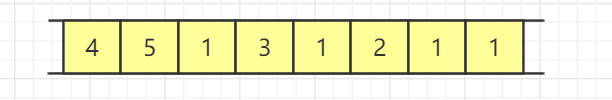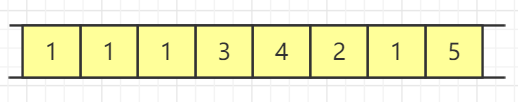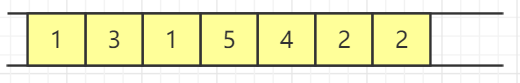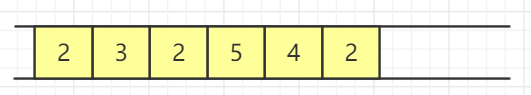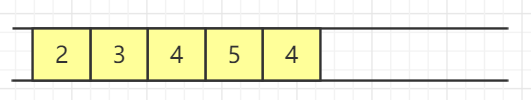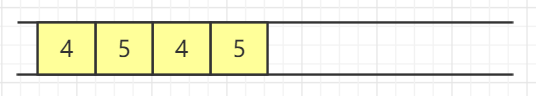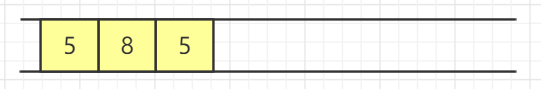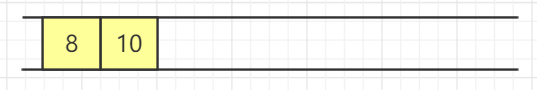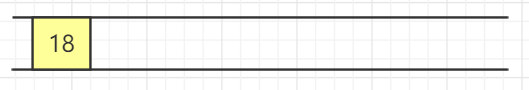## 代码实现

#include<iostream>
using namespace std;
void heapify(int a_heap[], int idx1, int idx2);
void creatHeap(int a_heap[], int n);
int main()
{
int a_heap;
int count;      //堆中剩余元素个数
int heap_top;      //暂时保存第一个堆顶
int money = 0;      //总费用

cin >> count;
for (int i = 1; i <= count; i++)
{
cin >> a_heap[i];
}
creatHeap(a_heap, count);    //建初堆
while (count != 1)
{
heap_top = a_heap;    //提取第一个最小花费
a_heap = a_heap[count--];
heapify(a_heap, 1, count);    //调整堆寻找第二个最小花费
a_heap += heap_top;    //提取第二个最小花费，将花费相加，入堆
money += a_heap;    //更新总花费
heapify(a_heap, 1, count);    // 调整堆，准备下一次拼接
}
cout << money;
return 0;
}

void heapify(int a_heap[], int idx1, int idx2)    //调整堆，细节见上文
{
int insert_node = a_heap[idx1];

for (int i = 2 * idx1; i <= idx2; i *= 2)
{
if (i < idx2 && a_heap[i] > a_heap[i + 1])
{
i++;
}
if (insert_node <= a_heap[i])
{
break;
}
a_heap[idx1] = a_heap[i];
idx1 = i;
}
a_heap[idx1] = insert_node;
}

void creatHeap(int a_heap[], int n)    //建初堆，细节见上文
{
for (int i = n / 2; i > 0; i--)
{
heapify(a_heap, i, n);
}
}


# 延伸阅读

posted @ 2020-05-24 16:24  乌漆WhiteMoon  阅读(1061)  评论(0编辑  收藏  举报